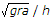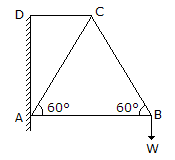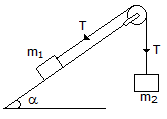Test: Engineering Mechanics - 3

# Test: Engineering Mechanics - 3

Test Description

## 25 Questions MCQ Test Additional Study Material for Mechanical Engineering | Test: Engineering Mechanics - 3

Test: Engineering Mechanics - 3 for Mechanical Engineering 2023 is part of Additional Study Material for Mechanical Engineering preparation. The Test: Engineering Mechanics - 3 questions and answers have been prepared according to the Mechanical Engineering exam syllabus.The Test: Engineering Mechanics - 3 MCQs are made for Mechanical Engineering 2023 Exam. Find important definitions, questions, notes, meanings, examples, exercises, MCQs and online tests for Test: Engineering Mechanics - 3 below.
Solutions of Test: Engineering Mechanics - 3 questions in English are available as part of our Additional Study Material for Mechanical Engineering for Mechanical Engineering & Test: Engineering Mechanics - 3 solutions in Hindi for Additional Study Material for Mechanical Engineering course. Download more important topics, notes, lectures and mock test series for Mechanical Engineering Exam by signing up for free. Attempt Test: Engineering Mechanics - 3 | 25 questions in 25 minutes | Mock test for Mechanical Engineering preparation | Free important questions MCQ to study Additional Study Material for Mechanical Engineering for Mechanical Engineering Exam | Download free PDF with solutions
 1 Crore+ students have signed up on EduRev. Have you?
Test: Engineering Mechanics - 3 - Question 1

### The point, through which the whole weight of the body acts, irrespective of its position, is known as

Test: Engineering Mechanics - 3 - Question 2

### The loss of kinetic energy during elastic impact is zero.

Test: Engineering Mechanics - 3 - Question 3

### The overturning of a vehicle on a level circular path can be avoided if the velocity of vehicle is __________.

Test: Engineering Mechanics - 3 - Question 4

Which of the following is a scalar quantity?

Test: Engineering Mechanics - 3 - Question 5

The rate of change of momentum is directly proportional to the impressed force, and takes place in the same direction in which the force acts. This statement is known as

Test: Engineering Mechanics - 3 - Question 6

In ideal machines, mechanical advantage is __________ velocity ratio.

Test: Engineering Mechanics - 3 - Question 7

The minimum force required to slide a body of weight W on a rough horizontal plane is

Test: Engineering Mechanics - 3 - Question 8

The moment of inertia of a square of side a about its diagonal is

Test: Engineering Mechanics - 3 - Question 9

The angular velocity (in rad / s) of a body rotating at N revolutions per minute is

Test: Engineering Mechanics - 3 - Question 10

The maximum efficiency of a lifting machine is

Test: Engineering Mechanics - 3 - Question 11

The centre of gravity of a semi-circle lies at a distance of __________ from its base measured along the vertical radius.

Test: Engineering Mechanics - 3 - Question 12

The process of finding out the resultant force is called __________ of forces.

Test: Engineering Mechanics - 3 - Question 13

A weight of 1000 N can be lifted by an effort of 80 N. If the velocity ratio is 20, the machine is

Test: Engineering Mechanics - 3 - Question 14

In a framed structure, as shown in the below figure, the force in the member BC isTest: Engineering Mechanics - 3 - Question 15

The potential energy of a vertically raised body is __________ the kinetic energy of a vertically falling body.

Test: Engineering Mechanics - 3 - Question 16

If a number of forces acting at a point be represented in magnitude and direction by the three sides of a triangle, taken in order, then the forces are not in equilibrium.

Test: Engineering Mechanics - 3 - Question 17

The periodic time of a particle with simple harmonic motion is __________ proportional to the angular velocity.

Test: Engineering Mechanics - 3 - Question 18

The motion of a particle round a fixed axis is

Test: Engineering Mechanics - 3 - Question 19

A block of mass m1, placed on an inclined smooth plane is connected by a light string passing over a smooth pulley to mass m2, which moves vertically downwards as shown in the below figure. The tension in the string isTest: Engineering Mechanics - 3 - Question 20

If a given force (or a given system of forces) acting on a body __________ the position of the body, but keeps it in equilibrium, then its effect is to produce internal stress in the body.

Test: Engineering Mechanics - 3 - Question 21

The principle of transmissibility of forces states that, when a force acts upon a body, its effect is

Test: Engineering Mechanics - 3 - Question 22

A differential pulley block has larger and smaller diameters of 100 mm and 80 mm respectively. Its velocity ratio is

Test: Engineering Mechanics - 3 - Question 23

If three forces acting at a point are represented in magnitude and direction by the three sides of a triangle, taken in order, then the forces are in equilibrium.

Test: Engineering Mechanics - 3 - Question 24

Newton's second law motion __________ a relation between force and mass of a moving body.

Test: Engineering Mechanics - 3 - Question 25

The periodic time of one oscillation for a simple pendulum is(where l = Length of the pendulum.)

## Additional Study Material for Mechanical Engineering

1 videos|30 docs|57 tests
Information about Test: Engineering Mechanics - 3 Page
In this test you can find the Exam questions for Test: Engineering Mechanics - 3 solved & explained in the simplest way possible. Besides giving Questions and answers for Test: Engineering Mechanics - 3, EduRev gives you an ample number of Online tests for practice

## Additional Study Material for Mechanical Engineering

1 videos|30 docs|57 tests(Scan QR code)